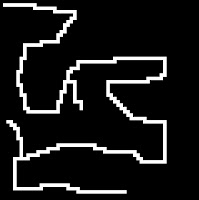## Friday, April 10, 2009

### How to find all the modes of a 3D probability distribution surface

A 3D probability distribution surface can represent the likelihood of certain events in a specific region where a higher point on the surface could mean it is more likely for the event to happen. For example, a 3D probability distribution surface created for a Wilderness Search and Rescue (WiSAR) operation, whether systematically or manually or with a hybrid, can show the searchers areas where it is more likely to find the missing person. The distribution map can be used to better allocate search resources and to generate flight paths for an Unmanned Aerial Vehicle (UAV).An example 3D probability distribution surface
Because different path-planning algorithms may be better suited for different probability distributions (I appeal to the No-Free-Lunch theorem), identifying the type of distribution beforehand can help us decide what algorithm to use for the path-planning task. In our decision process, we particularly care about how many modes the probability distribution has. So how can we automatically identify all the modes in a 3D probability distribution surface? Here I'll describe the algorithm we used.

In our case, the 3D probability distribution surface is represented by a matrix/table where each value represents the height of the point. You can think of this distribution as a gray-scale image where the gray value of each pixel represent the height of the point. And we use a Local Hill Climbing type algorithm with 8-connected neighbors.

1. Down sample the distribution
If the distribution map is very large, it might be a good idea to down sample the distribution to improve algorithm speed. We assume the surface is noise-free. If the surface is noisy, we can also smooth it with a Gaussian filter (think image processing).

2. Check for a uniform distribution (a flat surface)
It is a good idea to check if the probability distribution is a uniform distribution. Just check to see if all values in the matrix are identical. If a uniform distribution is identified, we know the distribution has 0 mode and we are done.

3. Local Hill Climbing with Memory
Start from the a point of the surface and then check its neighbors (8-connected). As soon as a neighbor with the same or better value is found, we "climb" to that point. The process is repeated until we reach a point (hilltop) where all neighbors have smaller values. As we "climb" and check neighbors, we mark all the points we visited along the way. And when we check neighbors, we only check points we have not visited before. This way we avoid finding a mode we had found before. Once we find a "mode", we can start from another unvisited point on the surface and do another Local Hill Climbing. Here I use quotes around the word mode because we are not sure if the "mode" we found is a real mode.

4. Make sure the "mode" we found is a real modeAn Even-Height Great Wall
The "mode" we found using Local Hill Climbing might not actually be a real mode. It might be right next to a mode previously found and have a lower value (because we only checked unvisited neighbors in the previous step). It might also be part of another flat-surface mode where the mode consists of multiple points with identical values (think of a hilltop that looks like a plateau or think of a ridge). Things get even more complicated with special distributions such as this one on the right. And the "mode" point we found might be connected to a previously found mode through other points with the same value (e.g, the "mode" point is the end point of the short branch in the middle of the image.

Therefore, we need to keep track of all points leading to the final "mode" point that have identical values and check all the visited neighbors of these points, making sure this flat surface is not part of a previously found mode. If these points make up a real new mode, we mark these points with a unique mode count id (e.g, mode 3). If they are only part of a previous found mode, we mark these points so (e.g., mode 2). If one of them is right next to a previously found mode but have lower value, we mark these points as non-mode points. This step is almost like performing a Connected-Component Labeling operation in Computer Vision.

At the end of the algorithm run, we will have a count of how many modes the probability distribution has and also a map with all the mode points marked. With the Even-Height Great Wall distribution, the map would look just like the image (white pixels marking mode points) with 1 mode. And within Milli-seconds, the algorithm can identify the 4 modes in the example 3D surface above.

That's it! If you ever need to do this for your projects, you now know how!Recursive functions work great for local hill climbing until you get a stack overflow.